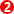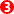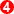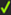﻿ MechDesigner Reference & User Interface > Dialog-Boxes > Dialog: Function-Block: Math

# Dialog: Function-Block: Math

Navigation:  MechDesigner Reference & User Interface > Dialog-Boxes >

# Dialog: Function-Block: Math

## Math FB

See also :Tutorial 18: Maths FB

Use a Math FB to add new math functions, add limits to motion-values, and combine data that might be useful for your modeling.

You can use a Maths FB to change the units of motion-values.

There is also a Calculator interface to help you select different functions.

### Open Math FB dialog-boxEdit the Math FB:

 1.Double-click the Math FB in the graphic-area - or - 1.

Math FB dialog-boxAdd Connectors; Edit Output Data Type   Add Input button- to add input-connectors to the Maths FB. •A new input-connector shows at its left each time you click Add Input. You need one input-connector for each variable or parameter in you equation.Add Output button- to add output-connectors to the Maths FB. •Each output-connector (and wire) has three data-channels, and •Click the drop-down box to select the Data-Type for all of the output-connectorsUpdate button- click this button to update ANY change in the dialog.Output Data-Type 1.Select the data-type in the Output Data-Type drop-down box. 2.Click the Update button before you add any equations.If you select Linear Coordinates , then ALL equations will be in Linear Units. If you have 4 output-connectors: •Data Output on Data-Channels 0, 3, 6, 9 is 'Dis', with units of m•Data Output on Data-Channels 1, 4, 7, 10 is 'Vel', with units of m/s•Data Output on Data-Channels 2, 5, 8, 11, is 'Acc', with units of m/s/sNotes: The Units inside the Maths FB are SI units: kilogram, meters, seconds, ... It is easy to use the buttons to add input and output-connectors. It is also easy to connect wires to the input and output-connectors. However, to be able to use the Maths FB you MUST understand Wire-Numbers/Input-Connectors and Data-ChannelsWire Numbers/input-connectors  and Data-Channels The image shows three Wires connected to three input-connectors. Each Wire has a three Data-Channels. Wire-Numbers - refer to the image above. Wire Numbers start at 0 •Input-Connector 1 = Wire Number 0 - connected to the TOP input-connector•Input-Connector 1 = Wire Number 1 - connected to the next input-connector, one down from the top.•Input-Connector 1 = Wire Number 2 - connected to the next input-connector down, again•Input-Connector 1 = ...Data-Channel Each wire has 3(three) data-channels. Each data-channel has a letter - p, v, and a (even if the data at the input is not a motion-value} •p = Data-Channel 1•v = Data-Channel 2•a = Data-Channel 3Each variable in an equation is identified by its: Data-Channel(Wire-Number) Example Entries in an Equation: p(0) : the p refers to Data-channel 1, and (0) refers to input-connector 1 a(2) : the a refers to Data-channel 3,  and (2) refers to input-connector 3 v(1) : the v refers to Data-channel 2,  and (1) refers to input-connector 2 How many input and output-connectors? By way of an example, to calculate Power from Torque × Angular Velocity P =  Ʈ . ω Torque(N.m.) × Angular Velocity (rad/sec) Torque data and Angular-Velocity data are the inputs and Power is the output. Thus, you need two input-connectors and one output-connector. To get Torque data: we must add a Force-Data FBto the model. To get Angular-Velocity data: the output from the Motion-Dimension FBis in the model. There is one output-connector when we add the Maths FB to the model.Set the Output Data Type to Power. Click UPDATE after we select the Output Data Type and before we add equations. The Maths FB uses SI units for all data at its input-connectors The equation in the Maths FB is:×p(0)*v(1) Wire-Number 0 (top), Channel 1 (p = Torque) × Wire-Number 1 (bottom wire), Channel 2 (v = Angular Velocity) SYNTAX and VALID Equations: Click the Update after you edit the equation to find if its syntax is correct or not correct. For example, the syntax is not correct if it does not have the correct number of parentheses. The reason is given as a message in the Feedback-Area. Theat the left of each equation shows whether its syntax is correct.### MORE ABOUT UNITS 'inside' the Maths FB

There are 4 possible sources of confusion with respect to 'units' when you use the Maths FB.

Data from Motion FBs

If you connect a wire from a Motion FB to the input of a Maths FB, set the Output Data Type IN THE MOTION FB to Linear or Rotary to indicate the SI units you want to apply to an equation in the Maths FB

SI Units

Inside the Maths FB, the units are always SI. Thus, data from a Motion FB

oIf the Motion FB is set to rotary units, and the output from it is 90º, then the value inside the Maths FB is 1.57radians.

oIf the Motion FB is set to linear units, and the output from it is 100mm, the value inside the Maths FB is 0.1m.

If you multiply these together with the Maths FB, the result 'inside' the Maths FB is 0.157 - of course.

#### Output motion-values and Coordinates

Even though the values internal to the Maths FB are SI, the units are converted back to units of the Machine Settings dialog at the output-connector, for the units you select in the Output Data-Type drop-down box.

For example: Machine Settings

If the units (inside the Maths FB) are set to Linear Coordinates, then 0.157 becomes 157mm at the output-connector when the units in the Machine Settings dialog-box are mm.

If the units (inside the Maths FB) are set to Rotary Coordinates, then 0.157 becomes at the output-connector when the units in the Machine Settings dialog-box are degrees.

Confusion between Linear Coordinates given to a Rocker, and a Rotary Coordinates given to a Slider

Rotary units connected to a Linear Slider - that is probably not intended

If you set the Output Data Types to Rotary Coordinates, then 0.157 becomes 0.157rads , which is . at the output, when the Machine Settings are degrees.

If you then connect the output to a Motion-Dimension FB to move a Slider, then the becomes 9mm.

Linear Units connected to a Rotary Rocker - that is probably not intended

If you set the Output Data Type to Linear Coordinates, then 0.157 becomes 157mm, when the Machine Settings are mm.
If you connect the output to a Motion-Dimension FB to move a Rocker, then the Rocker rotates 157º.

Maths FB CalculatorCalculator with special functions. Use these functions, when needed, with each equation. Click the Equation in the Maths FB, to make sure it is 'active', then the function in the Calculator. You will usually need to edit the equation again! The Calculator provides a few of the common mathematical functions. Note 1: When open and close brackets show within a function button, you must enter a value or an input variable. For example, Sin( ), you must enter at least one wire input. Sin(p(1)) will calculate the trigonometric 'Sine' of the position value of the second input-connector/ wire... Note 2: •p(0) is data on data-channel 1, at the top, or input-connector #1, •p(1) is data on data-channel 1, at input-connector #2, •p(2) is data on data-channel 1, at input-connector #3, ...Note 3: ALL data 'inside' the Maths FB are SI units. Calculator functions 1.Position, Velocity and Acceleration Values for an linear or angular input•P( ) ; V( ) ; A( )2.Standard Algebra•Arithmetic: +, –,  ∕ , *•Power: ^•Brackets: ( )3.Boolean•> : =1 if > , else 0•< : =1 if > , else 04.Trigonometry (all inputs in radians) •Standard: Sin( ), Cosine( ), Tangent( )•Hyperbolic: Sinh( ), Cosh( ), Tansh( )•Inverse: ArcSin( ), ArcCos( ) , ArcTan2( ; )5.Limit (input ; maximum value ; minimum value) : = if is 'less than maximum value' AND 'more than minimum value'. =   if is 'greater than maximum' =   if is 'less than minimum 6.OverLimit (input ; limit-value) : =  if is 'greater than limit-value' =  if is 'less than limit-value' 7.UnderLimit (input ; limit value) : =  if is 'less than limit-value' =  if is 'greater than limit-value' 8.XLimits (input ; maximum value ; minimum value) : = if is 'greater than maximum value' OR less than minimum value'. =   if is 'less than maximum' AND 'more than minimum-value =   if is 'greater than minimum-value AND 'less than maximum-value' 9.Numerical Keypad 10.1...9, 0, Π.11.Abs( ) 12.Mag( ; ) (assumes the two values are 'orthogonal' and uses Pythagoras to find the 'resultant')13.Sqrt( );14.DegToRad( )15.RadToDeg( )Example Maths FBExample 1: Rack Motion from a Pinion. Assume we want to drive a Rack from a Pinion. The Motion of a Rack is given by the Motion of the Pinion. The standard equations we should use are: •Rack Displacement = Pinion Radius * Pinion Rotation Angle of Pinion (D = R * Θ )•Rack Velocity = Pinion Radius * Pinion Angular Velocity of Pinion (V = R * ω )•Rack Acceleration = Pinion Radius * Angular Acceleration of Pinion (A = R * α )The units in the Maths FB are always SI Units. Linear Dimensions: If the Linear Units in the Machine Settings dialog are mm, then the dimension of a Part is in mm. If you change the Linear Units to m, then the dimension of the Part is in m (meters). For example, a dimension of 100 while the Machine Settings are set to millimeters, will become 0.1 if you change the units to meters in the Machine Settings. When you add a wire to a Maths FB, it will use the SI units m (meters). In this case you must multiply the Displacement equation by 1000. Angular Dimensions: If the units in the Machine Settings dialog are degs (degrees), and we measure the angle of a Part, it will show as degrees. However, when you connect a wire to a Maths FB, it will use the SI units for the degs (degrees), that is: rads (radians)Example 2: Epitrochoid / Hypotrochoid: When you have parametric equations, you can give the output for the X parameter to a horizontal slider, and the Y parameter to a piggyback orthogonal slider. If you enter the equations for •position only, then you can plot the Curve•position and velocity, then you can add a Cam with the Sliders (since a Cam needs position and velocity values)•position, velocity and acceleration, then you can plot Radius-of-Acceleration (since this needs all three motion derivatives)See Tutorial 18 for full details of this example. To make a Tool Translate at the end of a SCARA Robot. 1.Use Measurement FBs to measure the angles of the 'Upper Arm' and 'Lower Arm', relative to each other. 2.Add two Input-Connectors on the Maths FB3.Subtract the two angles to give an angle in the Maths FB4.Connect a wire at the output to the Motion-Dimension FB at the end of the SCARA to keep the tool fixed relative to the Base-Part.